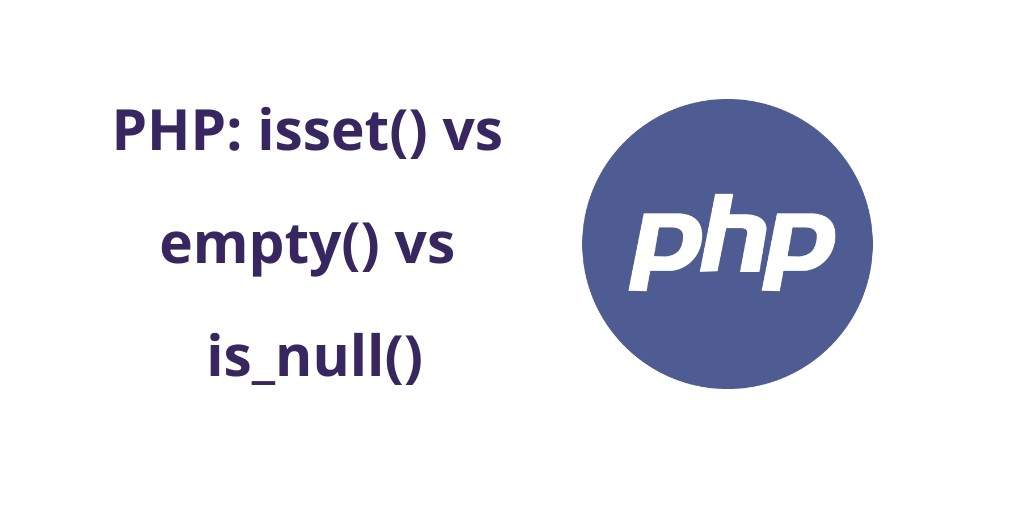Php 02-Sep-2021

### PHP: isset() vs empty() vs is_null()

In this tutorial, You will learn PHP empty() vs isset() vs is_null() function with its definition, syntax, require parameters and with examples. Also, we will show you the difference between is_null, empty() and isset().

### PHP | isset() vs empty() vs is_null()

This tutorial has purpose to explain an easy way, how to use php inbuilt functions isset(), empty(), & is_null(). And know difference the between isset() vs empty() vs is_null().

### PHP isset() function

Defination:- isset() is a inbuilt function of PHP. which is used to test/check if a variable value is set or not. This function returns the result as a boolean form (TRUE / FALSE).

Note:- If the variable value is set, this function will return the boolean value true. Otherwise, this function will return the boolean value false.

#### Syntax:

`isset(variable, ….);`

#### Parameters | isset() function

The PHP isset() function will accept multiple variables as shown in the above-given syntax of isset() function.

Note: You can pass more than one variable in this function, but this function will return true only if all of the variables are set.

#### Example PHP isset() function

Let’s take an example of a function isset(). Here, we have two variables. The first name is variable and the second is one name is variable1. We will first check and test it with isset() set the value in the variable. And also we will not set value of the second variable, we will check with an isset() function.

You can see the example below: “

<?php

// PHP code to check without set variable value
\$variable;

if( isset( \$variable ) ) {
print_r(" The variable value is set, checked by isset() ");
}else{
print_r(" The variable value is not set, checked by isset() ");
}

echo "<br>";

// PHP code to check with set variable value

\$variable2 = 'hello world';

if( isset( \$variable1 ) ) {
print_r(" The variable value is set, checked by isset() ");
}else{
print_r(" The variable value is not set, checked by isset() ");
}

?>

### PHP empty() function

Definition:- empty() is a inbuilt function of PHP. and commonly is to test/check if a given variable value is empty or not. This function returns the result as a boolean form (TRUE / FALSE).

Note:- If the variable value is not empty, this function will return the boolean value false. Otherwise, return the boolean value true.

#### Syntax:

`empty( \$variable )`

#### Parameters | empty() function

The empty() PHP function accepts one parameter only. You can see the syntax of this variable above.

#### Example of empty() function

In the example below, we have two variables, the first is num1 and the second is num2. We will first check and test it with empty() giving the value in the variable. And also we will set the null value of the second variable, we will check with an empty function.

You can see the example below:

<?php
// PHP Code to check first variable
\$variable;
if( empty( \$variable ) ) {
print_r(" The variable value is empty, checked by empty() ");
}else{
print_r(" The variable value is not empty, checked by empty() ");
}
echo "<br>";
// PHP Code to test variable with empty()

\$variable2 = 'PHP world!';

if( empty( \$variable2 ) ) {
print_r(" The variable value is empty");

}else{
print_r(" The variable value is not empty");

?>

### PHP is_null() function

Definition:- is_null() function is an inbuilt PHP function. Which is used to find / test whether a variable value is NULL or NOT. This function returns the result as a boolean form (TRUE / FALSE).

Note:- If the variable value is null, this function will return the boolean value true. Otherwise, this function returns the boolean value false.

#### Syntax:

`is_null( \$variable )`

#### Parameters | is_null() function

The is_null() PHP function accepts one parameter only. You can see the syntax of this variable above.

#### Example of is_null() function

In the example below, we have two variables, the first is variable and the second is variable1. We will set the null value of the variable, we will check with an is_null() function. Also, We will first check or test it with is_null() giving the value in the variable2.

You can see the example below:

<?php
// PHP Code or scritp to explain php is_null() function
\$variable = null;
if( is_null( \$variable ) ) {
print_r(" The variable value is null ");
}else{
print_r(" The variable value is not null");
}

echo "<br>";

// PHP Code to test variable with is_null()

\$variable2 = '10';

if( is_null( \$variable2 ) ) {
print_r(" The variable value is null ");
}else{
print_r(" The variable value is not null");
}
?>

### Uses of these functions

 Functions “” “apple” NULL FALSE 0 undefined empty() TRUE FALSE TRUE TRUE TRUE TRUE is_null() FALSE FALSE TRUE FALSE FALSE ERROR isset() TRUE TRUE FALSE TRUE TRUE FALSE